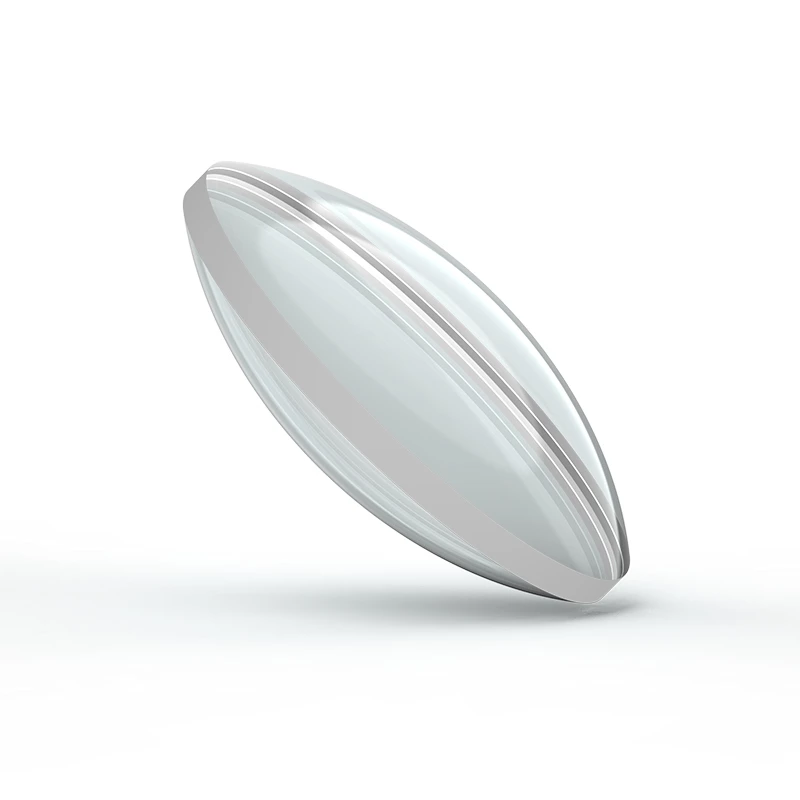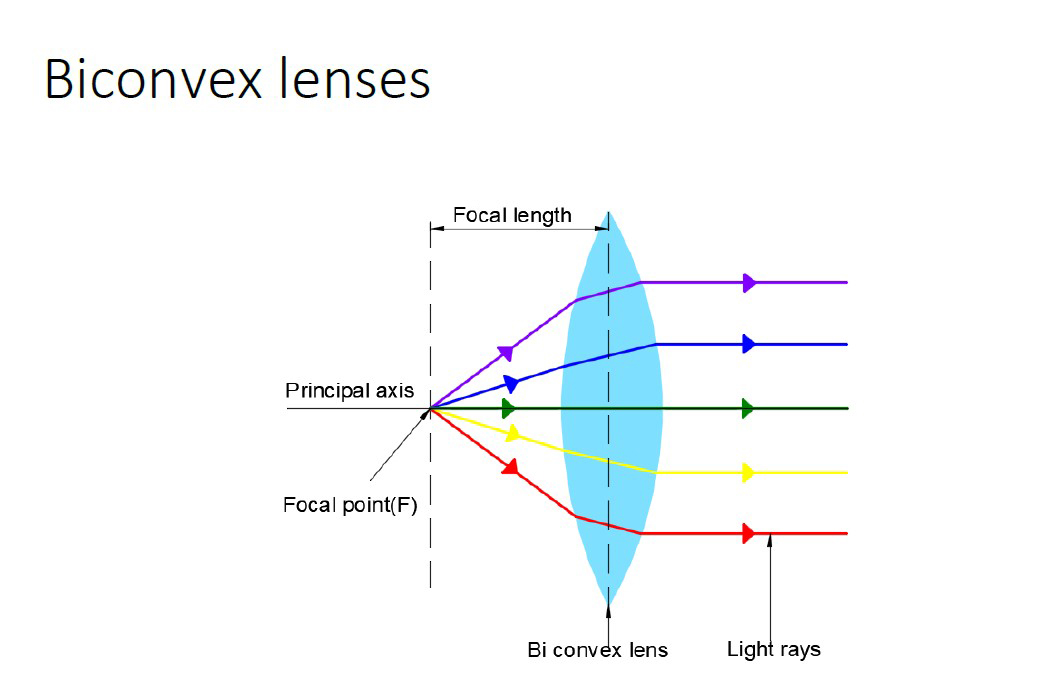Swati Talwar

HS-PS4-1

double convex lense uses

double convex lenses properties

difference between convex and double convex

# Double Convex Lenses Study Guide

Bi-convex (double-convex) lenses, like plano-convex lenses, have equal radii across both surfaces and focus parallel rays at the same place. As a rule of thumb, biconvex lenses work best with conjugate ratios from 5:1 to 1:5.

Table of content

# INTRODUCTION

A biconvex lens is a basic lens that consists of two convex surfaces in a spherical form with the same radius of curvature. A convex-convex lens is another name for this type of lens.

A biconvex lens is depicted below.Source

## DEFINITION OF DOUBLE CONVEX LENS

By blocking (bending) the rays twice, the biconvex lens additionally called a condensing lens, focuses on the divergent or unfocused rays of remote gadgets.

The eye of the human is an example of a double convex lens. In order to observe your surroundings, you bend the item`s ray of consciousness at the retina. Rays from remote gadgets require much less refraction.Source

## DIFFERENCE BETWEEN THE CONVEX AND DOUBLE CONVEX LENS

A plane convex lens is a plane on one side and convex on the other, whereas a biconvex or double convex lens, as used in magnifying glasses, is curved in a convex fashion on both sides of the glass.

## DOUBLE CONVEX LENSE PROPERTIES

A biconvex lens has the following characteristics:

• Biconvex lenses are referred to as having a focal length that is positive.
• Biconvex lenses have shorter focal lengths.
• Double convex lenses have the capability of combining incident light.
• They can generate both real and virtual images, and they can reduce spherical aberration while canceling coma and distortion by employing a conjugate ratio of unity.

Biconvex lenses have equal radii on both sides and are symmetrical in appearance. They're usually recommended for virtual imaging of real things, as well as a positive conjugate ratio of 0.2 to 5.

## DOUBLE CONVEX LENSE APPLICATIONS

These lenses can be used for a wide range of applications, some of which are listed below -

• Biconvex lenses are used as magnifying or condensing lenses.
• They can also be used as objectives or magnifiers.
• These are used in telescopes, monoculars, microscopes, binoculars, cameras, projectors, and other imaging equipment.
• In the case of a human eye, these are used to generate a virtual image, while in the case of photographic films or an optical sensor, they are used to create an actual image.
• You can also use these lenses as a burner.

# SUMMARY

• By refracting (bending) light rays twice, a double convex lens, also known as a converging lens, focuses divergent, or unfocused, light rays from a distant object.
• Biconvex lenses have equal radii on both sides and are symmetrical in appearance. They're usually recommended for virtual imaging of real things, as well as a positive conjugate ratio of 0.2 to 5.

## FAQs

Q. Where are double convex lenses used?

As magnifying or condensing lenses, biconvex lenses are utilized. Telescopes, monoculars, microscopes, binoculars, cameras, projectors, and other image equipment all use these. These lenses can also be used as a burner.

Q. What does a double convex lens do to an image?

The focal points at twice the focal length are utilized to locate objects and images.

Q. What is the difference between a convex lens and a double convex lens?

A plane convex lens is a plane on one side and convex on the other, whereas a biconvex or double convex lens, as used in magnifying glasses, is curved in a convex fashion on both sides of the glass.

We hope you enjoyed studying this lesson and learned something cool about Double Convex Lenses! Join our Discord community to get any questions you may have answered and to engage with other students just like you! We promise, it makes studying much more fun!😎

## REFERENCE

1. Biconvex Lens: https://byjus.com/physics/biconvex-lens/ Accessed 18th April 2022.
2. Bi-Convex Lens: https://www.newport.com/c/bi-convex-lenses#:~:text=Bi%2DConvex%20(Double%2DConvex,%3A1%20and%201%3A5. Accessed 18th April 2022.